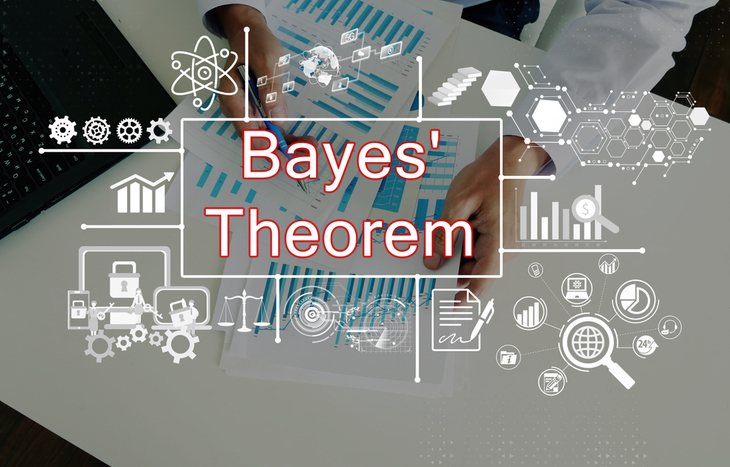The stock market is an ever-changing place. In fact, it’s changing every second of every day as prices go up and down, and new factors impact the trajectory of the market. It’s important for investors to adapt themselves to these changes. As they use new information to draw new conclusions, many investors are actually practicing high-level statistical analysis, called Bayes’ Theorem.

Named after polymath Thomas Bayes, Bayes’ Theorem is a way of looking at probability using historical information and new variables. For example, if ABC Company stock price drops three days in a row and the whole market is down on the fourth day, what is the likelihood that ABC Company is also down?

Many people use Bayes’ Theorem without realizing it, drawing simple conclusions in their head. But what many don’t realize is there’s actually a formula for computing the probability of certain situations, given known variables. Specifically, here’s how.## A Simplified Understanding of Bayes’ Theorem

It’s best to start with a simplified explanation of Bayes’ Theorem. To sum it up succinctly: prior probability, times a likelihood ratio, equals posterior probability. Confused already? Don’t worry, we’ll break it down.

• Prior probability is historical data you can use as a basis for predictions. For example, if ABC Company has performed negatively in December for 12 of the last 16 years, it’s reasonable to assume that there’s a 3:4 chance of it happening (75%).
• Likelihood ratio is the pure probability of an event. In general, ABC Company can perform positively or negatively in December. Those are the only options. There’s a 1:2 outcome for both of these scenarios (50%).
• Posterior probability is the probability of an outcome based on the likelihood of the event. If you know that ABC Company does poorly at a rate of 3:4 and there’s a 1:2 chance that it will perform positively or negatively, you can predict the outcome.

This is Bayes’ Theorem at work: using data you have to predict events with a relative degree of likelihood. The answer to the question “what’s the chance that ABC Company performs poorly next December?” is 75%. However, to understand the math behind it, let’s look at the actual theorem.

## The Formula for Bayes’ Theorem

There are several iterations of Bayes’ Theorem, depending on the number of variables involved. For now, let’s look at the simplest base version:

P(A|B) = (P(B|A) × P(A)) / P(B)

• A, B are events
• P(A|B) is the probability of A given B is true
• P(B|A) is the probability of B given A is true
• P(A), P(B) are the independent probabilities of A and B

This formula allows investors to take data that they know and use it to predict future events using probability ratios. The importance of this equation is that it shows both sides of a potential outcome and the probabilities associated with it.

## An Example of the Theorem

Let’s use our example from above: ABC Company’s year-end performance woes.

Say that you’re an investor with shares of ABC Company, and the stock price is on a tear lately. In fact, you bought at \$50. And over the course of three months, the stock reached \$100. It’s November and you’re thinking about taking profits. However, you don’t want to trim your position too early. You know it usually does poorly in December.

You can apply Bayes’ Theorem to help inform your decision to either hold or sell. For example, here are the variables using data from the last 16 years:

• The stock will perform well in December (A)
• Stock will perform poorly in December (B)
• The probability the stock will perform well is 1:4 (P(A|B))
• Probability the stock will perform poorly is 3:4 (P(B|A))
• The independent probabilities of good or poor performance are 1:2 (P(A), P(B))

Using the formula for Bayes’ Theorem results in this: ((3:4) × (1:2)) / (1:2). The outcome is 3:4 or 75%. If you’re a shareholder of ABC Company who wants to take profits, you should do it before December.

## When to Use Bayesian Statistics

Bayes’ Theorem has applications in just about every corner of the investing world. It’s not only used to predict the probability of stock price movements, it’s also useful for looking ahead at the effect of interest rate increases and the probability of dividend offerings. Determining posterior probability plays a role in deciphering how companies, markets and even other investors will behave given a set of prior data points.

Bayesian statistics goes far beyond investing. It’s practical and useful in virtually every aspect of life that requires us to make forward-thinking decisions. It’s impossible to tell the future, but it’s another thing entirely to predict it with relative accuracy. If you know how something behaves historically given a set of variables, you can reasonably predict how it might behave in the future given those same variables.

## Look at Finance Through the Lens of Probability

Timing plays a significant role in investing. In fact, this includes both short-term trades and long-term investments. To time entry or exit from a position takes forethought into the future behavior of that security. And while there’s no definitive future, there’s a statistical probability attached to different outcomes. Investors can use past behavior and the rate of certain instances to inform a forward-looking understanding of what’s to come.

The beauty of Bayes’ Theorem and Bayesian statistics is that they’re evolving. Every new piece of data contributes to the historical picture of probability. As time goes on and more data becomes available, the probability of future events becomes clearer. Investors, and anyone, really, who understands Bayes’ Theorem will find themselves feeling confident about the future ahead.